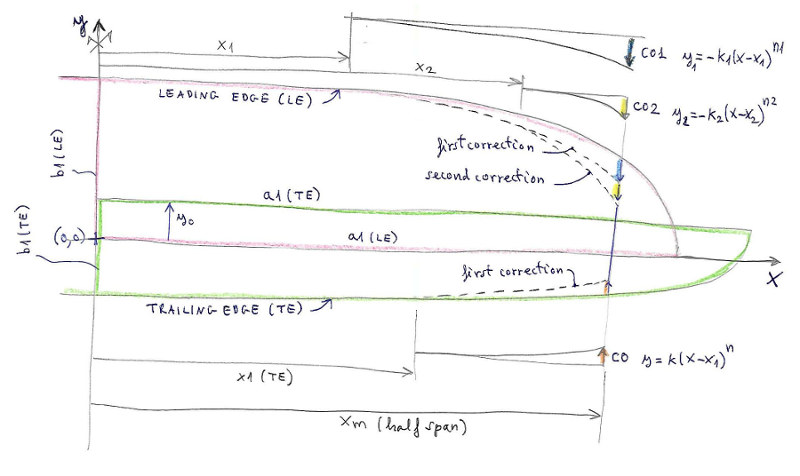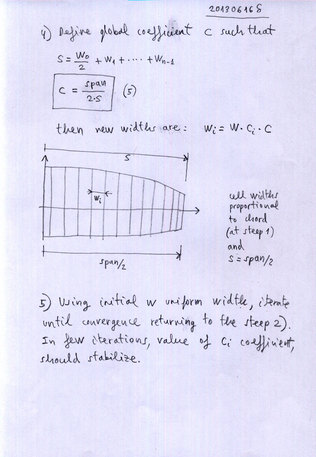[en] [fr] [ru]

LEPARAGLIDING 2.60/3.01
PRE-PROCESSOR OF GEOMETRY 1.5 (OPTIONAL)

GEOMETRY PRE-PROCESSOR for use with leparagliding.txt input file of LEparagliding 2.60/3.01
Version 1.0 "Kemerovo" (2013-06-16)
Version 1.1 "Kemerovo" (2013-06-24)
Version 1.2 "Gurzuf" (2015-09-05)
Version 1.3 "Utah" (2016-04-17)
Version 1.4 "Escaules" (2016-08-24)
Version 1.5 "Baldiri" (2018-12-07)

This preprocessor is an optional module (but very useful) for use with LEparagliding. Only serves to help generate the geometry matrix of the wing, which can also be generated by drawing directly into a CAD program or even manually with pencil and paper, as before. Its main application is in the definition of paragliders with many cells, where there is a lot of manual work definition. To generate complex geometries (different from normal) is always necessary manual labor, but the array generated can serve as a basis.

BASIC INSTRUCTIONS:

INPUT FILE:

Fill in the data file with the geometric parameters (
details below) that define the main curves of the wing, leading edge, trailing edge, and main vault.
Choose and define a distribution of cells along span.

OUTPUT FILES:

geometry.dxf --> DXF containig planview, and distribution of cells, main vault

geometry-out.txt --> TXT file containig matrix of geometry for use with LEparagliding 2.60 or 3.01 input file leparagliding.txt section 1. GEOMETRY
Auxiliar geometry matrix for use with LEparagliding 2.2:
Rib    x-rib      y-LE       y-TE       xp    z    beta      RP        Washin

SOURCE CODE:

pre-processor.f --> GNU Fortran source code :-) !

EXECUTABLE FILES:

a.exe (windows version, use with cygwin1.dll in the same directory)

a.out (GNU/Linux)

 Version 1.4 pre-processor.zip Version 1.5 pre-processor1.5.zip Only source code, and Linux. pre-1.5-w10-x64.zip Compiled for Windows 10 Intel 64bit pre-1.5-win32.zip Windows 32bit
If the .exe dot does not work on your computer, please contact the Laboratori and we will try to compile a version for you.
First try to compile the pre-processor.f file with Linux or using a Cygwin console.

DESCRIPTION OF A TYPICAL INPUT DATA FILE

Since version 1.5
some additional parameters have been added, for a more precise definition of the leading edge and the trailing edge.

Now the leading edge section looks like:

**********************************
**********************************
1
a1= 641.92
b1= 194.02
x1= 340
x2= 490
xm= 527
c01= 28
ex1= 2.8
c02= 12
ex2= 4.0

Explanation: The first "1" means defining leading edge type 1.The leading edge is defined by an ellipse of semiaxis a1 and b1 (red), centered at the point (0.0). Xm is half span. In versions 1.4 and earlier it is only possible to modify the ellipses with a parabolic correction (degree 2). Now it is possible to make two corrections with a generic curve of degree N. The first correction begins at the point x1 and allows a deflection c01, with a variation of degree ex1.
The second correction begins at the point x2 > x1 and allows a deflection c02, with a variation of degree ex2.

And the trailig edge section:

**********************************
* 2. Trailing edge parameters
**********************************
1
a1= 643.28
b1= 140.5
x1= 180
xm= 527
c0= -8.9
y0= 88.06
exp= 1.5

Explanation: The first "1" means defining trailing edge type 1.The trailing edge is defined by an ellipse of semiaxis a1 and b1 (green), centered at the point (0,y0). Xm is half span. In versions 1.4 and earlier it is only possible to modify the ellipses with a parabolic correction (degree 2). Now it is possible to make correction with a generic curve of degree N. The correction begins at the point x1 and allows a deflection c0, with a variation of degree exp.Leading edge and trailing edge definition and corrections in pre-processor version >= 1.5

It is mandatory to use at least one space between the sign "=" and the parameter.
Recommended units cm and degrees.
The others sections are defined as in version 1.4.

DESCRIPTION OF A TYPICAL INPUT DATA FILE pre-data.txt (version 1.4):

**********************************
LEPARAGLIDING
GEOMETRY PRE-PROCESSOR     v1.4
**********************************
gnu-A
**********************************
**********************************
a1= 609.68
b1= 223.58
x1= 291.5
xm= 539
c0= 40
**********************************
* 2. Trailing edge parameters
**********************************
a1= 636.925
b1= 127.97
x1= 352.77
xm= 539
c0= -3.834
y0= 42.22
**********************************
* 3. Vault
**********************************
2
640.56    20.35
480.47    21.367
229.50    18.925
99.26    28.349
**********************************
* 4. Cells distribution
**********************************
3
0.3
27

Sections 1. 2. and 3. change parameters acording figures below.

Section 3. Vault.
If first number is "1" then vault using ellipse and cosinus modification, indicate parameters a1, b1,
If first number is "2" then vault using four tangent circles. In four rows indicate radious and angle (deg).

Section 4. Cells distribution
- First number "3" indicates cell width proportional to chord
- Second number is a coefficient between "0.0" and "1.0". If coefficient is "0" then cell width is estrictly proportional to the chord, using iterative calculus. If coefficient is set to "1.0", then cell width is uniform. Use intermediate values as you need.
- Third number is the total cell number.
If first number is "4" then we use explicit width of each cell with automatic adjustement, if the sum not match the span (view examples).

pre-data.txt Example NOTES:The first step is to draw on a CAD program (or even manually with pencil and paper), the main curves of the wing: leading edge, trailing edge, vault, and then deduce the geometric parameters to insert in the input file pre-data.txt.The program includes a routine to automatically adjust the length of the vault to the length of the wing in planview (span). So if the length of the vault is introduced slightly larger or smaller than the total width of the wing, does not matter, because it is adjusted to the span=2*Xm. Then run the program: a.exe (WIndows 32-bit) or ./a.out (GNU/Linux) geometry.dxf file is just to confirm that the data entered are the desired Then open geometry-output.txt file and copy (and modify if necessary) the matrix of geometry for use with LEparagliding 2.2 input file (leparagliding.txt section 1. GEOMETRY) Rib    x-rib      y-LE       y-TE       xp    z    beta      RP        Washin

FIGURES: GEOMETRIC PARAMETERS DETAILS

Note: to complete the pre-data.txt file, you only need to know to identify what are the geometric parameters a1, b1, x1, xm, c, y0 ... indicated in the accompanying figures. The mathematical description is additional detail (just  to satisfy the curiosity of students of mathematics) as implemented in the Fortran source code of the program.

Main parameters for leading edge, trailing edge, and vault:

Alternative vault using four tangent circlesCell widths proporcional to chord and using control coefficient k from 0.0 (full proportional) to 1.0 (uniform width)index